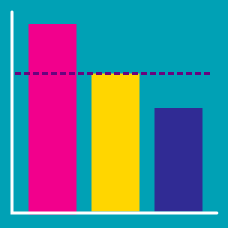Discrete Mathematics

Expected Value - Definition

What is the expected value of the following probability distribution: $P(X=1) = \frac{19}{40}, P(X=2) = \frac{21}{40} .$

There are seven balls of the same shape in a pocket, each labelled with an integer from 0 to 6. Danielle draws a ball out, takes the number written on it as $x,$ and puts the ball back in. Then she makes another draw, and takes the number on it as $y.$ If the numbers $x$ and $y$ satisfy $\sin\left(\frac{(x+y)}{6}\pi\right)=\frac{\sqrt{3}}{2}\ x,y\in[0,6]$ what is the conditional expectation of $x?$

Note: Every ball has a different number written on it, i.e. integers from 0 to 6 are all used once.

$X$ is a random variable taking on the values decided by the roll of a dice with numbers $1$ through $6$ written on it. What is the expected value of $X,$ or $E(X)?$

After throwing a dice with numbers 1 through 6 written on it, you get $4$ dollars for an odd number and $10$ dollars for an even number. Let random variable $X$ be the prize you get after throwing the dice, then what is the expected value of $X?$

What is the expected value of the following probability distribution: $P(X=1) = \frac{2}{25}, P(X=2) = \frac{13}{50}, P(X=3) = \frac{33}{50} .$

×